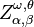# Issue:Compass-and-straightedge constructions in the intuitionistic fuzzy interpretational triangle: two new intuitionistic fuzzy modal operators

 shortcut http://ifigenia.org/wiki/issue:nifs/23/2/1-7
Title of paper: Compass-and-straightedge constructions in the intuitionistic fuzzy interpretational triangle: two new intuitionistic fuzzy modal operators
Author(s):
 Vassia Atanassova Department of Bioinformatics and Mathematical Modelling Institute of Biophysics and Biomedical Engineering Bulgarian Academy of Sciences, Acad. G. Bonchev Str., Bl. 105, Sofia 1113, Bulgaria vassia.atanassovagmail.com Lyubka Doukovska Intelligent Systems Department Institute of Information and Communication Technologies, Bulgarian Academy of Sciences, Acad. G. Bonchev str., Bl. 2, 1113 Sofia, Bulgaria doukovskaiit.bas.bg
Presented at: 21st International Conference on Intuitionistic Fuzzy Sets, 22–23 May 2017, Burgas, Bulgaria
Published in: "Notes on IFS", Volume 23, 2017, Number 2, pages 1—7
Abstract: The idea about the two new intuitionistic fuzzy modal operators, proposed here, was inspired by a review of the modal operators defined over intuitionistic fuzzy sets and the observation that graphically all of them are constructed by orthogonal projections. Here for the first time, we propose a new method of constructing two different modal operators, using a compass-andstraightedge construction, producing for each point from the intuitionistic fuzzy interpretational triangle, the two points onto the triangle’s hypothenuse that are respectively equidistant from the Truth and the Falsity as the point itself. The properties of these so-constructed new intuitionistic fuzzy operators are studied and formulated in two theorems.
Keywords: Intuitionistic fuzzy modal operator, Intuitionistic fuzzy interpretational triangle, Ruler-and-compass construction, InterCriteria Analysis.
AMS Classification: 03E72.
References:
1. Atanassov, K. (1989). Geometrical interpretation of the elements of the intuitionistic fuzzy objects, Preprint IM-MFAIS-1-89, Sofia, 1989, Reprinted: Int J Bioautomation. 2016, 20(S1), S27–S42.
2. Atanassov, K. (2017). Intuitionistic Fuzzy Logics. Springer, Cham.
3. Atanassov, K., Szmidt, E., & Kacprzyk, J. (2013). On intuitionistic fuzzy pairs, Notes on Intuitionistic Fuzzy Sets, 19(3), 1–13.
4. Atanassov, K. (2005). On one type of intuitionistic fuzzy modal operators. Notes on Intuitionistic Fuzzy Sets, 11(5), 24–28.
5. Atanassov, K. (2004). On the modal operators defined over intuitionistic fuzzy sets . Notes on Intuitionistic Fuzzy Sets, 10(1), 7–12.
6. Atanassov, K. (2006). The most general form of one type of intuitionistic fuzzy modal operators. Notes on Intuitionistic Fuzzy Sets, 12(2), 36–38.
7. Atanassov, K. (2008). The most general form of one type of intuitionistic fuzzy modal operators. Part 2 . Notes on Intuitionistic Fuzzy Sets, 14(1), 27–32.
8. Atanassov K. (1989). Two variants of intuitionistic fuzzy modal logics, Mathematical Foundations of Artificial Intelligence Seminar, Sofia, 1989, Preprint IM-MFAIS-3-89. Reprinted: Int J Bioautomation, 2016, 20(S1), S43–S54.
9. Atanassova, V. (2015). Interpretation in the intuitionistic fuzzy triangle of the results, obtained by the intercriteria analysis, Proc. of 16th World Congress of the International Fuzzy Systems Association (IFSA), 9th Conference of the European Society for Fuzzy Logic and Technology (EUSFLAT), 30. 06-03. 07. 2015, Gijon, Spain, 1369–1374.
10. Atanassova, V., Vardeva, I., Sotirova, E., & Doukovska, L. (2016). Traversing and ranking of elements of an intuitionistic fuzzy set in the intuitionistic fuzzy interpretation triangle, Novel Developments in Uncertainty Representation and Processing, Vol. 401, Advances in Intelligent Systems and Computing, Springer, 161–174.
11. Çuvalcioğlu, G. (2013). The extension of modal operators diagram, Notes on Intuitionistic Fuzzy Sets, 19(3), 56–61.
12. Çuvalcioğlu, G. (2007). Some properties of Eα,β operator, Advanced Studies on Contemporary Mathematics, 14(2), 305–310.
13. Çuvalcioğlu, G. (2010). Expand the modal operator diagram with$Z^{\omega, \theta}_{\alpha, \beta}$, Proc. Jangjeon Math. Soc., 13(3), 403–412.
14. Dencheva, K. (2004). Extension of intuitionistic fuzzy modal operators ⊞ and ☒. Proceedings of the Second Int. IEEE Conference on Intelligent Systems, Varna, June 22–24, 2004, Vol. 3, 21–22.
15. Marinov, E. (2013). π-ordering and index of indeterminacy for intuitionistic fuzzy sets. Proc. of 12th Int. Workshop on IFS and GN, IWIFSGN’13, SRI-PAS, Warsaw, 2014, 129–138.
16. De Miguel, L., Bustince, H., Fernandez, J., Induráin, E., Kolesárová, A., & Mesiar, R. (2016). Construction of Admissible Linear Orders for Interval-Valued Atanassov Intuitionistic Fuzzy Sets with an Application to Decision Making. Information Fusion, 27, 189197.
17. Vassilev, P., & Stoyanov, T. (2016). On a New Ordering between Intuitionistic Fuzzy Pairs. Proc. of 8th European Symposium on Computational Intelligence and Mathematics, Sofia, Bulgaria October 5th-8th,77-80
Citations:

The list of publications, citing this article may be empty or incomplete. If you can provide relevant data, please, write on the talk page.